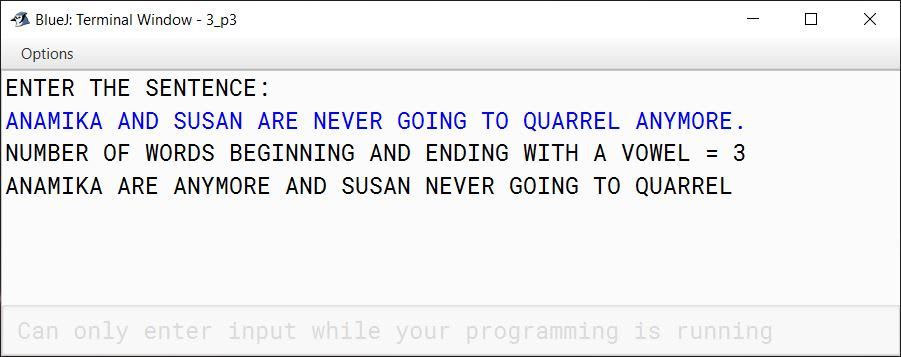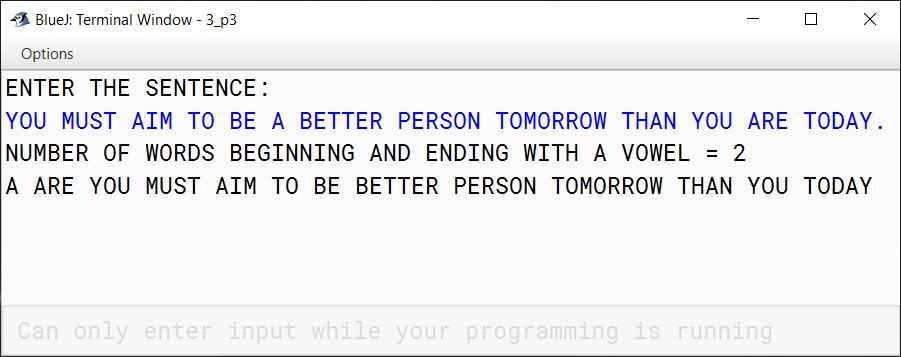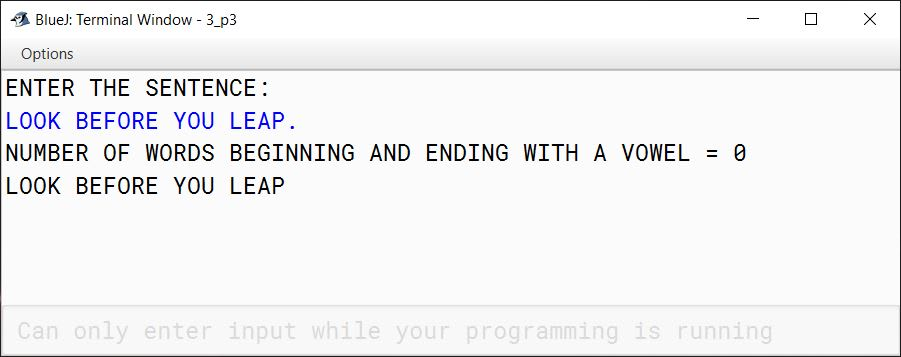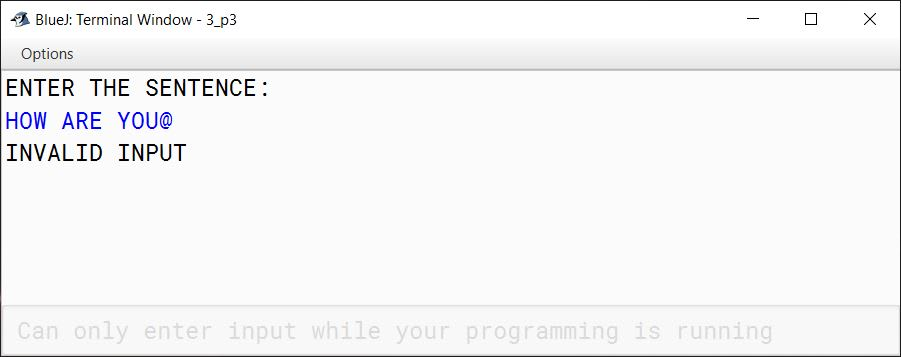# Solved 2016 Practical Paper ISC Computer Science

## Practical Questions

#### Question 1

A Circular Prime is a prime number that remains prime under cyclic shifts of its digits. When the leftmost digit is removed and replaced at the end of the remaining string of digits, the generated number is still prime. The process is repeated until the original number is reached again.

A number is said to be prime if it has only two factors 1 and itself.

Example:
131
311
113
Hence, 131 is a circular prime.

Accept a positive number N and check whether it is a circular prime or not. The new numbers formed after the shifting of the digits should also be displayed.

Test your program with the following data and some random data:

Example 1

INPUT:
N = 197

OUTPUT:
197
971
719
197 IS A CIRCULAR PRIME.

Example 2

INPUT:
N = 1193

OUTPUT:
1193
1931
9311
3119
1193 IS A CIRCULAR PRIME.

Example 3

INPUT:
N = 29

OUTPUT:
29
92
29 IS NOT A CIRCULAR PRIME.

##### Solution
```import java.util.Scanner;

public class CircularPrime
{
public static boolean isPrime(int num) {
int c = 0;

for (int i = 1; i <= num; i++) {
if (num % i == 0) {
c++;
}
}

return c == 2;
}

public static int getDigitCount(int num) {
int c = 0;

while (num != 0) {
c++;
num /= 10;
}

return c;
}

public static void main(String args[]) {
Scanner in = new Scanner(System.in);
System.out.print("ENTER INTEGER TO CHECK (N): ");
int n = in.nextInt();

if (n <= 0) {
System.out.println("INVALID INPUT");
return;
}

boolean isCircularPrime = true;
if (isPrime(n)) {
System.out.println(n);
int digitCount = getDigitCount(n);
int divisor = (int)(Math.pow(10, digitCount - 1));
int n2 = n;
for (int i = 1; i < digitCount; i++) {
int t1 = n2 / divisor;
int t2 = n2 % divisor;
n2 = t2 * 10 + t1;
System.out.println(n2);
if (!isPrime(n2)) {
isCircularPrime = false;
break;
}
}
}
else {
isCircularPrime = false;
}

if (isCircularPrime) {
System.out.println(n + " IS A CIRCULAR PRIME.");
}
else {
System.out.println(n + " IS NOT A CIRCULAR PRIME.");
}

}
}```
##### Output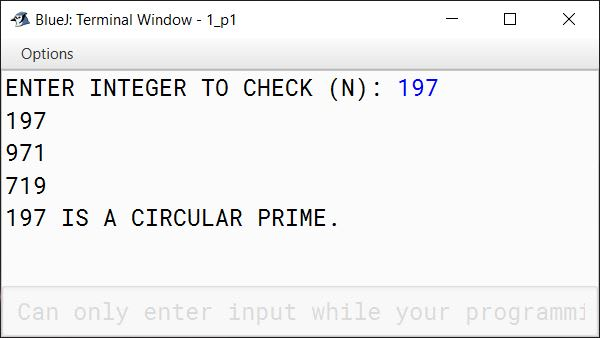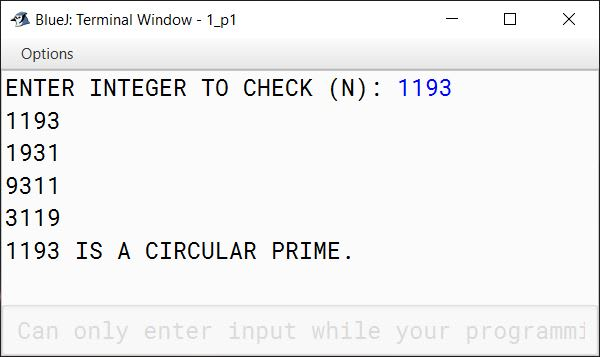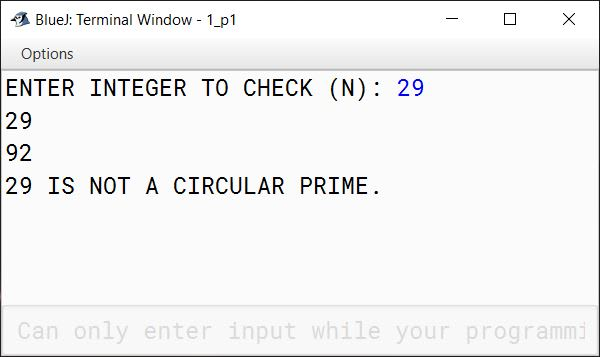#### Question 2

Write a program to declare a square matrix A[][] of order (M × M) where 'M' must be greater than 3 and less than 10. Allow the user to input positive integers into this matrix. Perform the following tasks on the matrix:

1. Sort the non-boundary elements in ascending order using any standard sorting technique and rearrange them in the matrix.
2. Calculate the sum of both the diagonals.
3. Display the original matrix, rearranged matrix and only the diagonal elements of the rearranged matrix with their sum.

Test your program for the following data and some random data:

Example 1

INPUT:
M = 4
9    2    1    5
8   13   8    4
15   6   3    11
7   12   23   8

OUTPUT:
ORIGINAL MATRIX
9    2    1    5
8   13   8    4
15   6   3    11
7   12   23   8
REARRANGED MATRIX
9    2    1    5
8    3    6    4
15  8   13  11
7   12   23   8
DIAGONAL ELEMENTS
9            5
3    6
8   13
7             8
SUM OF THE DIAGONAL ELEMENTS = 59

Example 2

INPUT:
M = 5
7    4    1    9    5
8    2    6   10   19
13  1    3    5    1
10  0    5   12   16
1    8   17   6    8

OUTPUT:
ORIGINAL MATRIX
7    4    1    9    5
8    2    6   10   19
13  1    3    5    1
10  0    5   12   16
1    8   17   6    8
REARRANGED MATRIX
7    4    1    9    5
8    0    1    2    19
13  3    5    5    1
10  6   10  12   16
1    8   17   6    8
DIAGONAL ELEMENTS
7                5
0        2
5
6       12
1                 8
SUM OF THE DIAGONAL ELEMENTS = 46

Example 3

INPUT:
M = 3

OUTPUT:
THE MATRIX SIZE IS OUT OF RANGE.

##### Solution
```import java.util.Scanner;

public class MatrixSort
{
public static void main(String args[]) {
Scanner in = new Scanner(System.in);
System.out.print("ENTER MATRIX SIZE (M): ");
int m = in.nextInt();

if (m <= 3 || m >= 10) {
System.out.println("THE MATRIX SIZE IS OUT OF RANGE.");
return;
}

int a[][] = new int[m][m];

System.out.println("ENTER ELEMENTS OF MATRIX");
for (int i = 0; i < m; i++) {
System.out.println("ENTER ROW " + (i+1) + ":");
for (int j = 0; j < m; j++) {
a[i][j] = in.nextInt();
/*
* Only positive integers
* should be enetered
*/
if (a[i][j] < 0) {
System.out.println("INVALID INPUT");
return;
}
}
}

System.out.println("ORIGINAL MATRIX");
printMatrix(a, m);

sortNonBoundaryMatrix(a, m);
System.out.println("REARRANGED MATRIX");
printMatrix(a, m);

computePrintDiagonalSum(a, m);
}

public static void sortNonBoundaryMatrix(int a[][], int m) {
/*
* To sort non-boundary elements of Matrix
* copy the non-boundary elements in a
* single dimensional array. Sort the
* single dimensional array and assign the
* sorted elements back in the 2D array at
* their respective positions
*/
int b[] = new int[(m - 2) * (m - 2)];
int k = 0;
for (int i = 1; i < m - 1; i++) {
for (int j = 1; j < m - 1; j++) {
b[k++] = a[i][j];
}
}

for (int i = 0; i < k - 1; i++) {
for (int j = 0; j < k - i - 1; j++) {
if (b[j] > b[j + 1]) {
int t = b[j];
b[j] = b[j+1];
b[j+1] = t;
}
}
}

k = 0;
for (int i = 1; i < m - 1; i++) {
for (int j = 1; j < m - 1; j++) {
a[i][j] = b[k++];
}
}
}

public static void computePrintDiagonalSum(int a[][], int m) {
int sum = 0;
System.out.println("DIAGONAL ELEMENTS");
for (int i = 0; i < m; i++) {
for (int j = 0; j < m; j++) {
if (i == j || i + j == m - 1) {
sum += a[i][j];
System.out.print(a[i][j] + "\t");
}
else {
System.out.print("\t");
}
}
System.out.println();
}
System.out.println("SUM OF THE DIAGONAL ELEMENTS = " + sum);
}

public static void printMatrix(int a[][], int m) {
for (int i = 0; i < m; i++) {
for (int j = 0; j < m; j++) {
System.out.print(a[i][j] + "\t");
}
System.out.println();
}
}
}```
##### Output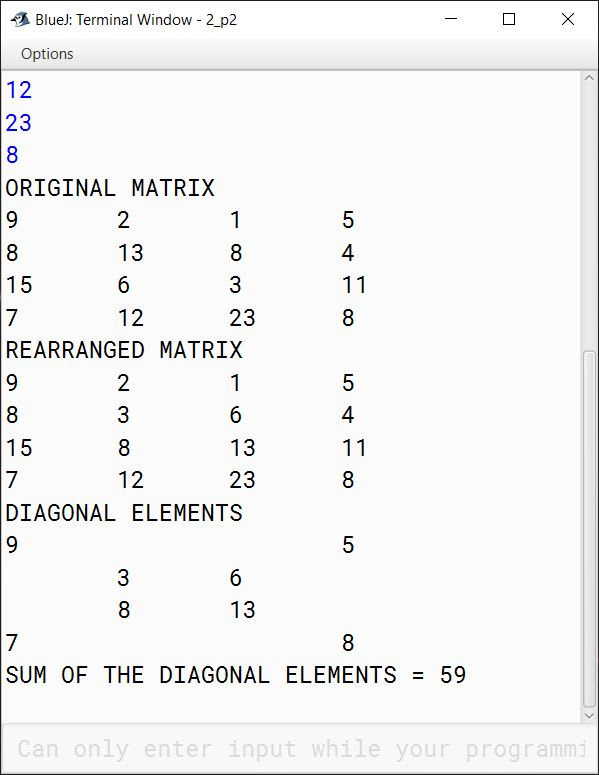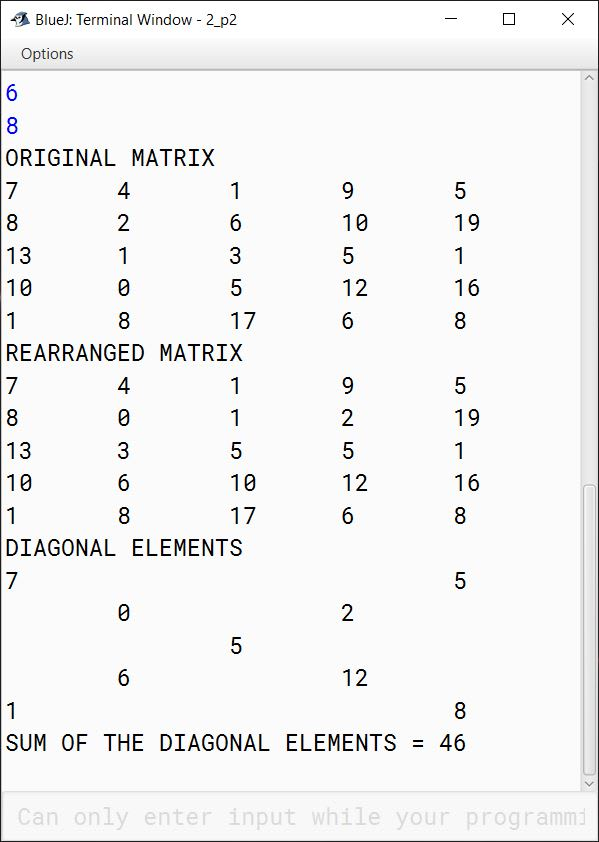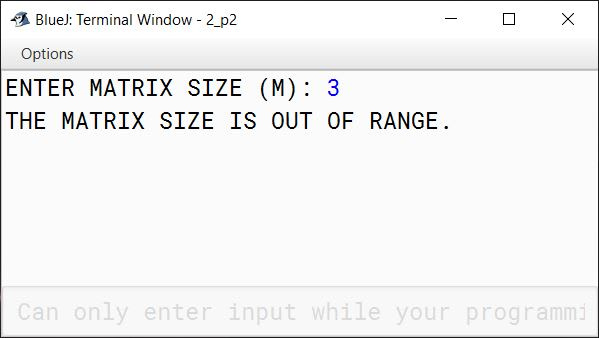#### Question 3

Write a program to accept a sentence which may be terminated by either '.', '?' or '!' only. The words may be separated by more than one blank space and are in UPPER CASE.

1. Find the number of words beginning and ending with a vowel.
2. Place the words which begin and end with a vowel at the beginning, followed by the remaining words as they occur in the sentence.

Test your program with the sample data and some random data:

Example 1

INPUT:
ANAMIKA AND SUSAN ARE NEVER GOING TO QUARREL ANYMORE.

OUTPUT:
NUMBER OF WORDS BEGINNING AND ENDING WITH A VOWEL = 3
ANAMIKA ARE ANYMORE AND SUSAN NEVER GOING TO QUARREL

Example 2

INPUT:
YOU MUST AIM TO BE A BETTER PERSON TOMORROW THAN YOU ARE TODAY.

OUTPUT:
NUMBER OF WORDS BEGINNING AND ENDING WITH A VOWEL = 2
A ARE YOU MUST AIM TO BE BETTER PERSON TOMORROW THAN YOU TODAY

Example 3

INPUT:
LOOK BEFORE YOU LEAP.

OUTPUT:
NUMBER OF WORDS BEGINNING AND ENDING WITH A VOWEL = 0
LOOK BEFORE YOU LEAP

Example 4

INPUT:
HOW ARE YOU@

OUTPUT:
INVALID INPUT

##### Solution
```import java.util.*;

public class VowelWord
{
public static void main(String args[]) {
Scanner in = new Scanner(System.in);
System.out.println("ENTER THE SENTENCE:");
String ipStr = in.nextLine().trim().toUpperCase();
int len = ipStr.length();

char lastChar = ipStr.charAt(len - 1);
if (lastChar != '.'
&& lastChar != '?'
&& lastChar != '!') {
System.out.println("INVALID INPUT");
return;
}

String str = ipStr.substring(0, len - 1);

StringTokenizer st = new StringTokenizer(str);
StringBuffer sbVowel = new StringBuffer();
StringBuffer sb = new StringBuffer();
int c = 0;

while (st.hasMoreTokens()) {
String word = st.nextToken();
int wordLen = word.length();
if (isVowel(word.charAt(0))
&& isVowel(word.charAt(wordLen - 1))) {
c++;
sbVowel.append(word);
sbVowel.append(" ");
}
else {
sb.append(word);
sb.append(" ");
}
}

String newStr = sbVowel.toString() + sb.toString();

System.out.println("NUMBER OF WORDS BEGINNING AND ENDING WITH A VOWEL = " + c);
System.out.println(newStr);
}

public static boolean isVowel(char ch) {
ch = Character.toUpperCase(ch);
boolean ret = false;
if (ch == 'A'
|| ch == 'E'
|| ch == 'I'
|| ch == 'O'
|| ch == 'U')
ret = true;

return ret;
}
}```
##### Output